Share

Books Shortlist
Your shortlist is empty

# RD Sharma solutions for Class 9 Mathematics chapter 14 - Quadrilaterals

## Chapter 14 - Quadrilaterals

#### Page 0

Three angles of a quadrilateral are respectively equal to 110°, 50° and 40°. Find its fourth angle

In a quadrilateral ABCD, the angles A, B, C and D are in the ratio 1 : 2 : 4 : 5. Find the measure of each angles of the quadrilateral

In a quadrilateral ABCD, CO and DO are the bisectors of ∠C and ∠D respectively. Prove that

∠COD = 1/2 (∠A+ ∠B).

The angles of a quadrilateral are in the ratio 3 : 5 : 9 : Find all the angles of the quadrilateral.

#### Page 0

Two opposite angles of a parallelogram are (3x – 2)° and (50 – x)°. Find the measure of each angle of the parallelogram .

If an angle of a parallelogram is two-third of its adjacent angle, find the angles of the parallelogram .

Find the measure of all the angles of a parallelogram, if one angle is 24° less than twice the smallest angle

The perimeter of a parallelogram is 22 cm .  If the longer side measures 6.5 cm what is the measure of the shorter side?

In a parallelogram ABCD, ∠D = 135°, determine the measures of ∠A and ∠B

ABCD is a parallelogram in which ∠A = 70°. Compute ∠B, ∠C and ∠D .

In Fig., below, ABCD is a parallelogram in which ∠A = 60°. If the bisectors of ∠A and ∠B meet at P, prove that AD = DP, PC = BC and DC = 2AD.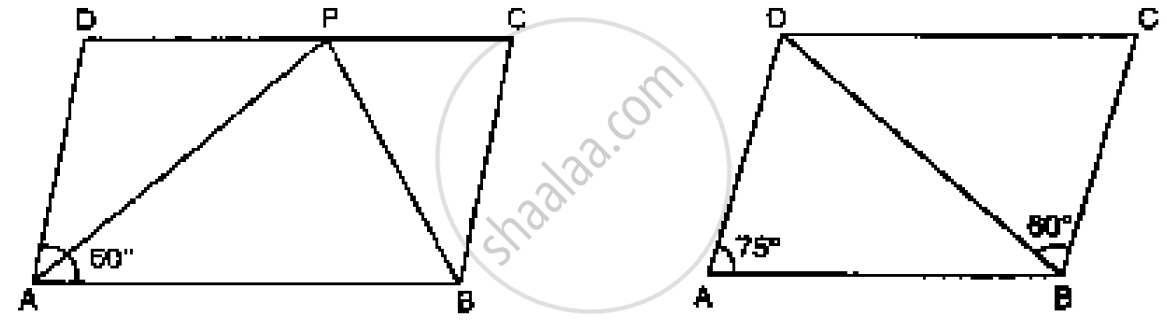In Fig. below, ABCD is a parallelogram in which ∠DAB = 75° and ∠DBC = 60°. Compute

In below fig. ABCD is a parallelogram and E is the mid-point of side B If DE and AB when produced meet at F, prove that AF = 2AB.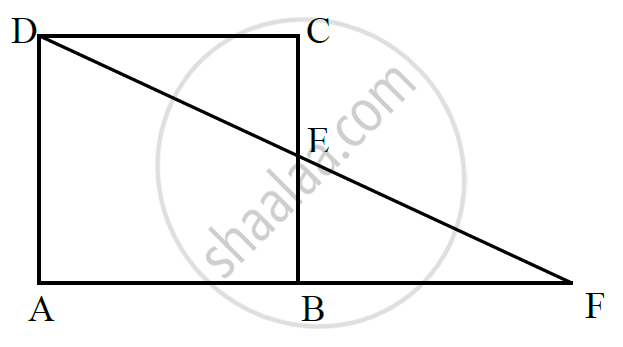The following statement are true  and false .

In a parallelogram, the diagonals are equal

The following statement are true  and false.

In a parallelogram, the diagonals bisect each other.

The following statement are true  and false .

In a parallelogram, the diagonals intersect each other at right angles .

The following statement are true  and false .

In any quadrilateral, if a pair of opposite sides is equal, it is a parallelogram.

The following statement are true  and false .

If all the angles of a quadrilateral are equal, it is a parallelogram .

The following statement are true  and false .

If three sides of a quadrilateral are equal, it is a parallelogram .

The following statement are true  and false .

If three angles of a quadrilateral are equal, it is a parallelogram .

The following statement are true  and false .

If all the sides of a quadrilateral are equal it is a parallelogram.

#### Page 0

In a parallelogram ABCD, determine the sum of angles ∠C and ∠D .

In a parallelogram ABCD, if ∠B = 135°, determine the measures of its other angles .

ABCD is a square. AC and BD intersect at O. State the measure of ∠AOB.

ABCD is a rectangle with ∠ABD = 40°. Determine ∠DBC .

The sides AB and CD of a parallelogram ABCD are bisected at E and F. Prove that EBFD is a parallelogram.

P and Q are the points of trisection of the diagonal BD of a parallelogram AB Prove that CQ is parallel to AP. Prove also that AC bisects PQ.

ABCD is a square E, F, G and H are points on AB, BC, CD and DA respectively, such that AE = BF = CG = DH. Prove that EFGH is a square.

ABCD is a rhombus, EABF is a straight line such that EA = AB = BF. Prove that ED and FC when produced meet at right angles

ABCD is a parallelogram, AD is produced to E so that DE = DC and EC produced meets AB produced in F. Prove that BF = BC.

#### Page 0

In a ∆ABC, D, E and F are, respectively, the mid-points of BC, CA and AB. If the lengths of side AB, BC and CA are 7 cm, 8 cm and 9 cm, respectively, find the perimeter of ∆DEF.

In a triangle ∠ABC, ∠A = 50°, ∠B = 60° and ∠C = 70°. Find the measures of the angles of

the triangle formed by joining the mid-points of the sides of this triangle.

In a triangle, P, Q and R are the mid-points of sides BC, CA and AB respectively. If AC =
21 cm, BC = 29 cm and AB = 30 cm, find the perimeter of the quadrilateral ARPQ.

In a ΔABC median AD is produced to X such that AD = DX. Prove that ABXC is a
parallelogram.

In a ΔABC, E and F are the mid-points of AC and AB respectively. The altitude AP to BC
intersects FE at Q. Prove that AQ = QP.

In a ΔABC, BM and CN are perpendiculars from B and C respectively on any line passing
through A. If L is the mid-point of BC, prove that ML = NL.

In Fig. below, triangle ABC is right-angled at B. Given that AB = 9 cm, AC = 15 cm and D,
E are the mid-points of the sides AB and AC respectively, calculate
(i) The length of BC (ii) The area of ΔADE.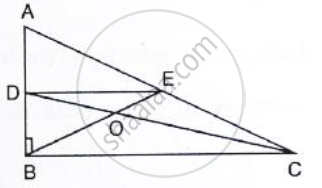In Fig. below, M, N and P are the mid-points of AB, AC and BC respectively. If MN = 3 cm, NP = 3.5 cm and MP = 2.5 cm, calculate BC, AB and AC.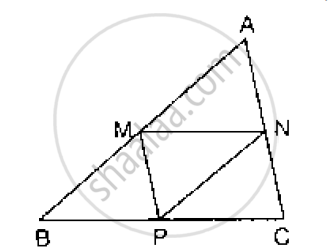ABC is a triangle and through A, B, C lines are drawn parallel to BC, CA and AB respectively
intersecting at P, Q and R. Prove that the perimeter of ΔPQR is double the perimeter of
ΔABC

In Fig. below, BE ⊥ AC. AD is any line from A to BC intersecting BE in H. P, Q and R are
respectively the mid-points of AH, AB and BC. Prove that ∠PQR = 90°.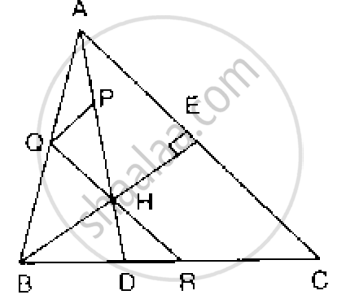In Fig. below, AB = AC and CP || BA and AP is the bisector of exterior ∠CAD of ΔABC.
Prove that (i) ∠PAC = ∠BCA (ii) ABCP is a parallelogram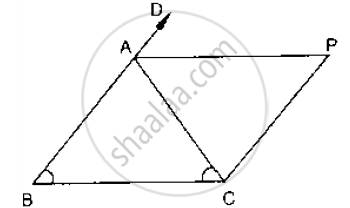ABCD is a kite having AB = AD and BC = CD. Prove that the figure formed by joining the
mid-points of the sides, in order, is a rectangle.

ABC is a triang D is a point on AB such that AD = 1/4 AB and E is a point on AC such that AE = 1/4 AC. Prove that DE = 1/4 BC.

In below Fig, ABCD is a parallelogram in which P is the mid-point of DC and Q is a point on AC such that CQ = 1/4 AC. If PQ produced meets BC at R, prove that R is a mid-point of BC.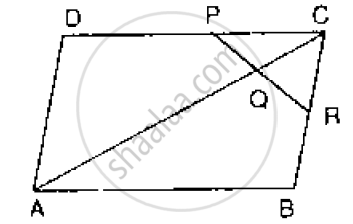In the below Fig, ABCD and PQRC are rectangles and Q is the mid-point of Prove thaT

i) DP = PC (ii) PR = 1/2 AC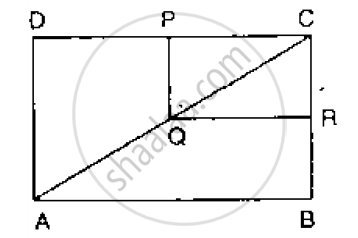ABCD is a parallelogram, E and F are the mid-points of AB and CD respectively. GH is any line intersecting AD, EF and BC at G, P and H respectively. Prove that GP = PH

BM and CN are perpendiculars to a line passing through the vertex A of a triangle ABC. If
L is the mid-point of BC, prove that LM = LN.

Show that the line segments joining the mid-points of the opposite sides of a quadrilateral
bisect each other.

Fill in the blank to make the following statement correct

The triangle formed by joining the mid-points of the sides of an isosceles triangle is

Fill in the blank to make the following statement correct:

The triangle formed by joining the mid-points of the sides of a right triangle is

Fill in the blank to make the following statement correct:

The figure formed by joining the mid-points of consecutive sides of a quadrilateral is

## RD Sharma solutions for Class 9 Mathematics chapter 14 - Quadrilaterals

RD Sharma solutions for Class 9 Maths chapter 14 (Quadrilaterals) include all questions with solution and detail explanation. This will clear students doubts about any question and improve application skills while preparing for board exams. The detailed, step-by-step solutions will help you understand the concepts better and clear your confusions, if any. Shaalaa.com has the CBSE Mathematics for Class 9 by R D Sharma (2018-19 Session) solutions in a manner that help students grasp basic concepts better and faster.

Further, we at shaalaa.com are providing such solutions so that students can prepare for written exams. RD Sharma textbook solutions can be a core help for self-study and acts as a perfect self-help guidance for students.

Concepts covered in Class 9 Mathematics chapter 14 Quadrilaterals are The Mid-point Theorem, Another Condition for a Quadrilateral to Be a Parallelogram, Properties of a Parallelogram, Types of Quadrilaterals, Angle Sum Property of a Quadrilateral, Concept of Quadrilaterals.

Using RD Sharma Class 9 solutions Quadrilaterals exercise by students are an easy way to prepare for the exams, as they involve solutions arranged chapter-wise also page wise. The questions involved in RD Sharma Solutions are important questions that can be asked in the final exam. Maximum students of CBSE Class 9 prefer RD Sharma Textbook Solutions to score more in exam.

Get the free view of chapter 14 Quadrilaterals Class 9 extra questions for Maths and can use shaalaa.com to keep it handy for your exam preparation

S